Join the forum, it's quick and easy

Would you like to react to this message? Create an account in a few clicks or log in to continue.

# [討論]我有一個LISP可以根據Y軸增量，給予高程標示，但我想改成X軸...

4 posters##[討論]我有一個LISP可以根據Y軸增量，給予高程標示，但我想改成X軸...

https://gph.is/g/4V8bVvo

DCDD：

`;; free lisp from cadviet.com;;; this lisp was downloaded from http://www.cadviet.com/forum/index.php?showtopic=56086&pid=171947&st=0&#entry171947;;;;;;  https://www.youtube.com/watch?v=3-i4i1p3cwM(defun c:dcdd (/ lstSS txtstr p1 p2 listname txt txt1 ss)(vl-load-com)(defun acet-ss-to-list (ss / n e l)  (setq n (sslength ss))  (while (setq e (ssname ss (setq n (1- n)))) (setq l (cons e l))  ));Gan gia tri goc(if (not k0) (setq k0 1));;gan gia tri goc(setq k (getreal (strcat "\nDrawing Ratio:1/" (rtos k0 2 0) "")));Nhap ty le ban ve(if (not k) (setq k k0) (setq k0 k))  (defun dowith(lstSS / lstSS en str)(cond  ((setq en  (car (vl-remove-if-not '(lambda(x)(wcmatch (cdadr (entget x))"*TEXT")) lstSS)))(setq str (acet-dxf 1 (entget en)) en (vlax-ename->vla-object en)))  ((setq en (car (vl-remove-if-not '(lambda(x)(and (wcmatch (cdadr (entget x))"INSERT")(= (acet-dxf 66 (entget x)) 1))) lstSS)))   (setq str (vla-get-textstring (setq en(car (vlax-invoke (vlax-ename->vla-object en) 'GetAttributes)))))  ))(cons en str))(grtext -1 "Edit By Nguy\U+1EC5n Ng\U+1ECDc S\U+01A1n")(setq  lstSS (acet-ss-to-list (setq ss (ssget)))  obj (car (setq en (dowith lstSS)))  str (cdr en)  p1 (getpoint "\nBase Point:")  eL (entlast) oDz (getvar "Dimzin"))(setvar "DIMZIN" 0)(while (setq p2 (getpoint p1 "\nNext Point:"))(command "copy" ss "" p1 p2)(while (setq EL (entnext EL)) (setq Listname (cons EL Listname)))(setq  Txt1 (car (dowith listName))  eL (entlast))(Ktra)(setvar "cecolor" "bylayer")  (vla-put-textstring txt1(strcat (cond ((> (setq num (+ (atof str) (/ (- (cadr p2)(cadr p1)) k))) 0) "0K+") ((= num 0) "%%p") (T "")   )(rtos num  2 2));So chu so dau dau ;))(setvar "DIMZIN" oDZ));Tim va tao moi Layer(defun ktra ()(if (not (tblsearch "layer" "Caodo")) (command "-LAYER" "m" "Caodo" "c" 1 "Caodo" "" ) (setvar "clayer" "Caodo" )`

CPT:

`(defun c:cpt (/ ss a b dis eg)  (setq ss (vl-remove-if 'listp (mapcar 'cadr (ssnamex (ssget '((0 . "*TEXT"))))))a (getpoint "\nBase Point：")  )  (setvar 'cmdecho 0)    (while (setq b (getpoint a  "\nNext Point："))    (setq dis (distance a b))    (princ (strcat "" (rtos dis)))    (foreach v ss      (command "copy" v "" "non" a "non" b)      (setq  eg (entget (entlast)))      (entmod (subst (cons 1 (rtos (+ dis (atof (cdr (assoc 1 eg)))))) (assoc 1 eg) eg))    )  )  (setvar 'cmdecho 1) (princ))`edmondsforum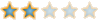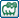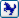##回復： [討論]我有一個LISP可以根據Y軸增量，給予高程標示，但我想改成X軸...

Atsai 在 2021-08-20, 19:28 作了第 1 次修改Atsai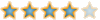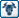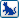yifeng.h likes this post

##回復： [討論]我有一個LISP可以根據Y軸增量，給予高程標示，但我想改成X軸...yifeng.h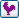##我也想要感謝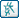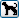##回復： [討論]我有一個LISP可以根據Y軸增量，給予高程標示，但我想改成X軸...Atsai